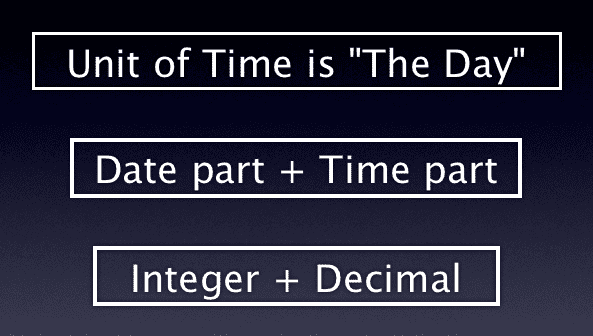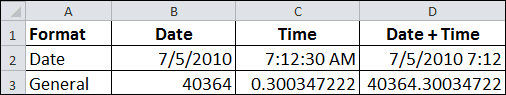# Date and Time Calculation in Excel

The unit of time in Excel is the Day. By using the NOW Function to show both date and time, you can see the underlying serial number by changing the cell format to General.

This serial number is composed of two parts: the date part is the integer and the time part is the decimal.The Date serial number is an integer value based upon a Date System, either Windows or Mac. The Time serial number is represented as a decimal fraction because time is considered a portion of a day.

## The Date and Time Calculator (Excel)

In the table below I entered a date in cell B2, a time in C2, and a formula in D2 that adds the two together. Excel automatically formatted this cell using a m/d/yyyy hh:mm format. I changed the row 3 cell formatting to General so you can see the serial number values. This is the function for time calculator in Excel.You can clearly see that Date is a integer value, Time is a decimal fraction, and the Date/Time format has both together in one number.

## Date and Time Calculations

In Excel, date serial number 1 is Jan 1, 1900 for the Windows Date System. The date serial number 40364 represents July 5, 2010 because it’s 40,363 days after Jan 1, 1900. These date serial numbers are used in calculations by Excel.

Time is a decimal value from zero (0) to 0.99999999, representing times from 0:00:00 (midnight) to 23:59:59 (11:59:59 pm).

Think about time values as the number of seconds past 12:00 AM divided by the number of seconds in a day, 86,400. Obviously this can be simplified if using only hours or minutes. For example, the time value for 6:00 AM is 0.25, which is 6hrs divided by 24hrs.

The time shown above (7:12:30 AM) has a decimal value of 0.300347222. The calculation is:

• 7 hours = 7 hrs x 60 min/hr x 60 sec/min = 25,200 seconds
• 12 minutes = 12 min x 60 sec/min = 720 seconds
• Total seconds = 25,200 + 720 + 30 = 25,950
• Decimal value = 25,950 / 86,400 = 0.300347222

Change the cell formatting to Time and you will see 7:12:30 AM (or your local Time setting format).

Tip: When subtracting two different times not in the same day, the integer portion of the serial number must be used or the calculation will be invalid.## 78 thoughts on “Date and Time Calculation in Excel”

1.Santhosh Shetty

I need to convert 5.45 PM time in 24Hr format.

Pls help!..

Many Regards
Santhosh Shetty

1.Gregory

You need to change the cell formatting. In cell A1 type in 5:45 AM, then in cell B1 type in the formula =A1 which will give you two cells with 5:45 PM. Select cell B1 and use the keyboard shortcut Ctrl+1 ( or CMD+1 on a Mac) to bring up the Format Cells dialog box. Click on the Number tab and select Time in the Category pane. You should see a few formats that don’t have an AM/PM, which you should select and click OK. (My choice was 13:30 which may or may not match what you will see.) YOu should now see 17:45 in cell B1.

Another way to show the 24 hour format is to change the cell formatting by using the Custom format and type in h:mm for hours and minutes.

2.Santhosh Shetty

Dear Gregory,
Wish you & your family a very happy & prosperous new year – 2013.

Thanks for the rply. Its working in the case time is mentioned in 5:45 PM format. But my time value is in the format 5.45 PM (.).

Best Regards
Santhosh Shetty!.

3.Aravind

Hi,

I want to calculate the numbers hours spent for a particular work.
I have the date and time in each cell as mentioned below
(3/18/2013 9:38) and (3/20/2013 12:20)
when i do minus both i.e {(3/18/2013 9:38)-(3/20/2013 12:20)} the answer should get be 51 hours 42 mints, but in excel cell it is display’s as 2:41:37.

mean it only considering the single day hours.

Help me out pls……..

4.Enayat

Dear Aravind,
There are many useful methods but the one I just tried with the result what you exactly wanted is below:
Pretend your start-time is located in Cell A1 and End-Time in B1.
A1>>> 3/18/2013 9:38:00 AM
B1>>>3/20/2013 12:20:00 PM
Formula: =(DAYS360(A1,B1)*24+1)+HOUR(MOD(B1-A1,1))&”:”&MINUTE(MOD(B1-A1,1))
First you need to find the number of days by function Days360 and Multiply it by 24Hours to find exact Hours based on number of days. Note: From 18th to 20th the result will be 2 days, while it should be three days e.g (18th,19th and 20th) therefore it should be +1. I think there is no need to explain the rest of the formula.. at a glance you can pick the meaning of the formula.
Hope you find it helpful,else, there are many respectful experts to guide you properly.
Regards,
Enayat

1.Siti

hi, Enayat,

I try to use your solution for Aravind problem due to I am also facing the same problem.However it does not work.

Currently I am facing the problemto calculate overtime for working shift people which is Overtime start on :
eg :

01 Apr 2013 9:30 pm until 1:30 am 02 April 2013 = 4 hours
How to calculate the total hours with different date.

5.sahan

I need to find a was to capture the date and time difference.
Eg: 01/04/2013 8:00am – 02/04/2013 09:00 i need to find the difference also don’t want to capture the full day. just the business hours which is 8am-5pm.
So the answer im looking for is 10Hurs. any way i can find a formula.

1.Gregory

Assume 1/4/13 8:00 is in cell A2 and 2/4/13 9:00 is in cell B2. The first and last day you would want to find the hours worked. So 17:00 – 8:00 for day 1 and 9:00 – 8:00 for the last day, which is 9 hours and 1 hour respectively. The number of weekdays is found by using the NETWORKDAYS function, which counts only weekdays, but you have to subtract the first and last day because we already calculated hours for those days. The rest of the weekdays are calculated at 10 hours for each day per the numbers you gave. A formula to calculate all that is:

=(((NETWORKDAYS(A2,B2)-2)*10)+((TIME(17,0,0)-MOD(A2,1)+MOD(B2,1)-TIME(8,0,0))*24))

The tricky part is to convert the first and last days hours from a time format to decimal hours, which is done by multiplying by 24 hours.

Hope this helps.

6.Sridipto

HI,

Need a help.

I need to get a formula so that I can get the difference of two dates and times..

Suppose, 5/1/2013 in A column
and 18:32 in column B;
again 5/2/2013 in C column and 20:26 in D column

I need to get the time difference of the above..(Like column A and Column B- Column C and Column D)

1.Gregory

In cell E1 enter the formula =(C1+D1)-(A1+B1) and then change the cell formatting to a custom time of [h]:mm where you need to have the square brackets around the hour symbol h to allow Excel to accumulate time past 24 hours. The result is 25:54 and is the total duration hours between those two dates/times.

7.Prashant

Hello, am using the =NOW() function and the column format is 12/24/2012 9:30 PM… but what I want is when i use the function i want the date to stay and the time initalize to 5:00 AM… need help!!!

Appreciate it!!!

1.Gregory

Use the following formula

=Today() + 5/24

and format your cell to a Date/time format.

8.Ramoen

Hi,
I was looking for something more simple, but can’t figure it out…
a certain time + a certain time frame = new time
e.g. 08:00 + 2.5 hours = 10:30

1.Ramoen